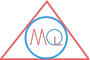# List of Symbols Used in Arithmetic

## Symbols used in Arithmetic

$$+$$Addition$$8 + 4$$
$$-$$Subtraction$$8 – 4$$
$$\div$$Division$$8 \div 4$$
$$\times$$Multiplication$$8 \times 4$$
$$\%$$Percentage$$8 \% 4$$
$$\gt$$Greater than$$8 \gt 4$$
$$\lt$$Less than$$4 \lt 8$$
$$\geq$$Greater than equal to$$a \geq b$$
$$\leq$$Less than equal to$$b \leq a$$
$$=$$equal to$$6 = 6$$
$$\neq$$does not equal to$$8 \neq 4$$
$$\approx$$Approximately equal to$$8.786 \approx 8.79$$
$$\pm$$Plus minus$$\pm 2$$
$$\equiv$$Equivalent to$$a \equiv b$$